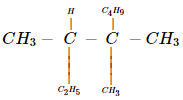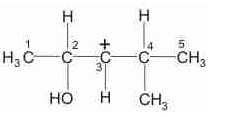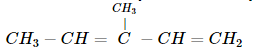Courses

# WBJEE Chemistry Test - 9

## 40 Questions MCQ Test WBJEE Sample Papers, Section Wise & Full Mock Tests | WBJEE Chemistry Test - 9

Description
This mock test of WBJEE Chemistry Test - 9 for JEE helps you for every JEE entrance exam. This contains 40 Multiple Choice Questions for JEE WBJEE Chemistry Test - 9 (mcq) to study with solutions a complete question bank. The solved questions answers in this WBJEE Chemistry Test - 9 quiz give you a good mix of easy questions and tough questions. JEE students definitely take this WBJEE Chemistry Test - 9 exercise for a better result in the exam. You can find other WBJEE Chemistry Test - 9 extra questions, long questions & short questions for JEE on EduRev as well by searching above.
QUESTION: 1

Solution:
QUESTION: 2

Solution:
QUESTION: 3

### Reductive ozonolysis of benzene produces

Solution:
QUESTION: 4
In India at the occasion of marriages, the fire works are used, which of the following gives green flame?
Solution:
QUESTION: 5
Nessler's reagent is
Solution:
QUESTION: 6

The uncertainty in the position of an electron (mass 9.1x 10-28g) moving with a velocity of 3.0 x 104 cm s-1 accurate up to 0.011%, will be

Solution:
QUESTION: 7
Which of the following does not show electromeric effect?
Solution:
QUESTION: 8
The number of molecules of ATP produced in the lipid metabolism of a molecule of palmitic acid is
Solution:
QUESTION: 9
In which of the following molecules/ions are all the bonds not equal?
Solution:
QUESTION: 10

The enthalpy of hydrogenation of cyclohexene is -119.5 KJ mol-1 if resonance energy of benzene is -150.4 KJ mol-1,its enthalpy of hydrogenation would be

Solution:
QUESTION: 11
Chemical equilibrium is dynamic in nature, because
Solution: The two opposite processes, forward reaction and reverse reaction, keep on occurring in it all the time but at exactly equal rates (or equal speeds)
A chemical reaction does not stop after reaching the equilibrium state, only the rate of the forward and the reverse reactions become equal at the equilibrium state
QUESTION: 12

For a reaction at 300 K, enthalpy and entropy changes are -11.5 x 103 J mol-1 and -105J K-1 mol-1 respectively. What is the change in Gibbs free energy?

Solution:

ΔG = ΔH - TΔS
= -(115 x 10)3 - [300 (-105)]
= 11500 - (- 31500) = 20000 J mol-1
= 20 kJ mol-1

QUESTION: 13
The rate of a chemical reaction
Solution:
QUESTION: 14
An antipyretic is
Solution:
QUESTION: 15

IUPAC name ofis

Solution:
QUESTION: 16

The number of unpaired electrons calculated in [ Co(NH3)6]3+  and [ CoF6 ]3- are

Solution:
QUESTION: 17
Caesium oxide is expected to be
Solution:
QUESTION: 18
Which one of the following indicates the value of the gas constant R ?
Solution:
QUESTION: 19
Which of the following is an electron deficient compound?
Solution:
QUESTION: 20

The electronic configuration of four different elements is given below. Identify the group IV element among these

Solution:
QUESTION: 21
Which one has the lowest boiling point?
Solution:
QUESTION: 22
Metal hydrides on treatment water gives
Solution:
QUESTION: 23
Which of the following is used for nuclear reaction?
Solution:
QUESTION: 24
The strongest base among the following is
Solution:
QUESTION: 25
Which one of the following pairs of compounds are functional isomers?
Solution:
QUESTION: 26
Which of the following is an example of synthetic polymer?
Solution:
QUESTION: 27
The compound is used in the manufacture of terylene is
Solution:
QUESTION: 28
If molecular weight of KMnO₄ is M, then its equivalent weight in acidic medium would be
Solution:
QUESTION: 29
The Miller indices of a plane having intercepts 2a, 2b, ∞ are
Solution:
QUESTION: 30
Ionic addition of bromine to cis-2-butene yields
Solution:
QUESTION: 31

The number of stereoisomers possible for a compound of the molecular formula
CH3  ― CH ═ CH ― CH (OH)   ― Me is

Solution:
QUESTION: 32

In the following carbocation, H/CH3 that is most likely to migrate to the positively charged carbon isSolution:
QUESTION: 33

Which set of products is expected on reductive ozonolysis of the following diolefin?Solution:
QUESTION: 34

The IUPAC name of neo-pentane is

Solution:
QUESTION: 35

The major product (70%-80%) of reaction between m-dinitrobenzene with (NH₄)₂ Sx is

Solution:
QUESTION: 36

Which statement are correct about osmotic pressure (P) volume (V) and temperature (T) ?

Solution:
QUESTION: 37

Which statement(s) about cathode rays is/are correct?

Solution:
QUESTION: 38

Which of following compounds on hydrolysis produce HCl ?

Solution:
QUESTION: 39

Which of the following statement(s) is (are) wrong?

Solution:
QUESTION: 40

Be and Al resembles in

Solution: